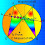## Thursday, June 23, 2011

### Problem 629: Triangle, Perpendicular to Sides, Intersecting Lines, Altitude

Geometry Problem
Level: Mathematics Education, High School, Honors Geometry, College.

Click the figure below to see the complete problem 629.1.analytic geometry ??
let be
A(0,0)B(a,b)C(c,0)D(0,d)E(c,e)
line AE:y=ex/c
line DC:y=-dx/c+d
line DB:y=(b-d)x/a+d
line BE:y=(e-b)(x-c)/(c-a)+e
point F: xF=-acd/(bc-cd-ea) yF=-aed/(bc-cd-ea)
2.